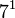# Groups of order 336

## Contents

See pages on algebraic structures of order 336| See pages on groups of a particular order

## Statistics at a glance

### Factorization and useful forms

336 has prime factors 2, 3, and 7, and prime factorization:$\! 336 = 2^4 \cdot 3^1 \cdot 7^1 = 16 \cdot 3 \cdot 7$

Other useful expressions for the number are:$\! 336 = 7^3 - 7 = 2(2^3 - 1)(2^3 - 2)(2^3 - 2^2)$

### Group counts

Quantity Value List/comment
Total number of groups up to isomorphism 228
Total number of abelian groups (i.e., finite abelian groups) up to isomorphism 5 (number of abelian groups of order$2^4$) times (number of abelian groups of order$3^1$) times (number of abelian groups of order$7^1$) =$5 \times 1 \times 1 = 5$. See classification of finite abelian groups and structure theorem for finitely generated abelian groups.
Total number of nilpotent groups (i.e., finite nilpotent groups) up to isomorphism 14 (number of groups of order 16) times (number of groups of order 3) times (number of groups of order 7) =$5 \times 1 \times 1 = 5$. See number of nilpotent groups equals product of number of groups of order each maximal prime power divisor, which in turn follows from equivalence of definitions of finite nilpotent group.
Total number of solvable groups (i.e., finite solvable groups) up to isomorphism 225 See note on non-solvable groups
Number of non-solvable groups up to isomorphism 3 there are only three non-solvable groups: special linear group:SL(2,7) (ID: (336,114)), projective general linear group:PGL(2,7) (ID: (336,208)), direct product of PSL(3,2) and Z2 (ID: (336,209))
All of these have composition factors PSL(3,2) and cyclic group:Z2.
Number of simple groups up to isomorphism 0
Number of quasisimple groups up to isomorphism 1 special linear group:SL(2,7) (ID: (336,114))
Number of almost simple groups up to isomorphism 1 projective general linear group:PGL(2,7) (ID: (336,208))
Number of almost quasisimple groups up to isomorphism 2 special linear group:SL(2,7) (ID: (336,114)), projective general linear group:PGL(2,7) (ID: (336,208))
Number of perfect groups up to isomorphism 1 special linear group:SL(2,7) (ID: (336,114))

## GAP implementation

The order 336 is part of GAP's SmallGroup library. Hence, any group of order 336 can be constructed using the SmallGroup function by specifying its group ID. Also, IdGroup is available, so the group ID of any group of this order can be queried.

Further, the collection of all groups of order 336 can be accessed as a list using GAP's AllSmallGroups function.

Here is GAP's summary information about how it stores groups of this order, accessed using GAP's SmallGroupsInformation function:

gap> SmallGroupsInformation(336);

There are 228 groups of order 336.
They are sorted by their Frattini factors.
1 has Frattini factor [ 42, 1 ].
2 has Frattini factor [ 42, 2 ].
3 has Frattini factor [ 42, 3 ].
4 has Frattini factor [ 42, 4 ].
5 has Frattini factor [ 42, 5 ].
6 has Frattini factor [ 42, 6 ].
7 - 22 have Frattini factor [ 84, 7 ].
23 - 47 have Frattini factor [ 84, 8 ].
48 - 55 have Frattini factor [ 84, 9 ].
56 has Frattini factor [ 84, 10 ].
57 has Frattini factor [ 84, 11 ].
58 - 73 have Frattini factor [ 84, 12 ].
74 - 89 have Frattini factor [ 84, 13 ].
90 - 105 have Frattini factor [ 84, 14 ].
106 - 113 have Frattini factor [ 84, 15 ].
114 has Frattini factor [ 168, 42 ].
115 - 117 have Frattini factor [ 168, 45 ].
118 - 120 have Frattini factor [ 168, 46 ].
121 - 130 have Frattini factor [ 168, 47 ].
131 - 133 have Frattini factor [ 168, 48 ].
134 - 136 have Frattini factor [ 168, 49 ].
137 - 163 have Frattini factor [ 168, 50 ].
164 - 167 have Frattini factor [ 168, 51 ].
168 - 170 have Frattini factor [ 168, 52 ].
171 - 173 have Frattini factor [ 168, 53 ].
174 - 183 have Frattini factor [ 168, 54 ].
184 - 193 have Frattini factor [ 168, 55 ].
194 - 203 have Frattini factor [ 168, 56 ].
204 - 207 have Frattini factor [ 168, 57 ].
208 - 228 have trivial Frattini subgroup.

For the selection functions the values of the following attributes
are precomputed and stored:
IsAbelian, IsNilpotentGroup, IsSupersolvableGroup, IsSolvableGroup,
LGLength, FrattinifactorSize and FrattinifactorId.

This size belongs to layer 2 of the SmallGroups library.
IdSmallGroup is available for this size.